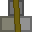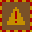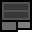# Account 220022

{0E0F} Clearing Bank - US Freeport II
Clearing Bank withdrawals

## BalanceMetal: 296s8 = 4744Explosives: 119s0 = 1904RC Turrets: 86

## Transfer

 PIN Receiver ReferenceMetal 16 × +Explosives 16 × +RC Turrets

## Create new account token

PIN:

New accounts cost 2 stacks (32 units) metal. You must give the token directly to the other player. Do not create the account yourself and give them the details. If you are found to do this, your account will be closed and all your items taken. You are responsible for ensuring the player you provide a new account token to knows how to use dredbank properly. If many players are found who don't know how to use dredbank properly, and they all got their accounts from one player, there may be a punishment against the player who provided their accounts.

## Transactions

Type Counterparty Account Reference NumberMetalExplosivesRC Turrets
Received 984526 0 1s0 = 16 0s0 = 0 0
Received 984526 0 2s0 = 32 0s0 = 0 0
Received 984526 0 30s0 = 480 0s0 = 0 0
Received 984526 0 10s0 = 160 0s0 = 0 0
Received 803203 666 39s0 = 624 0s0 = 0 0
Received 789542 0 16s8 = 264 0s0 = 0 0
Received 803203 6644 0s0 = 0 0s0 = 0 36
Received 803203 6644 0s0 = 0 23s0 = 368 0
Received 803203 6644 36s0 = 576 0s0 = 0 0
Received 452468 220022 0s0 = 0 0s0 = 0 14
Received 803203 13 10s0 = 160 0s0 = 0 0
Received 803203 12 10s0 = 160 0s0 = 0 0
Received 876411 0 0s0 = 0 0s0 = 0 1
Received 373306 9991 1s0 = 16 0s0 = 0 0
Received 837562 144 0s0 = 0 0s0 = 0 10
Received 837562 333 0s0 = 0 0s0 = 0 1
Received 298456 8383 16s0 = 256 10s0 = 160 0
Received 837562 88392 0s0 = 0 0s0 = 0 4
Received 632031 8833 0s0 = 0 0s0 = 0 20
Received 777188 92949698 60s0 = 960 0s0 = 0 0
Received 837562 9944 4s0 = 64 86s0 = 1376 0
Received 837562 1200 1s0 = 16 0s0 = 0 0
Received 837562 565 20s0 = 320 0s0 = 0 0
Received 837562 8844 20s0 = 320 0s0 = 0 0
Received 738995 1451 20s0 = 320 0s0 = 0 0